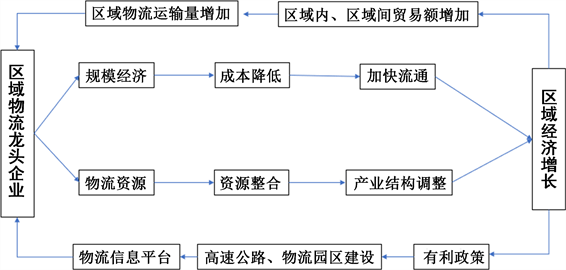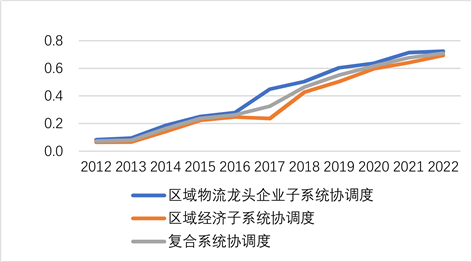#### 期刊菜单

Collaborative Development of Regional Logistics Leading Enterprises and Regional Economy Based on Synergy Degree Model—A Case Study of Jiangxi Province
DOI: 10.12677/MSE.2023.121006, PDF, HTML, XML, 下载: 25  浏览: 63

Abstract: The complex system composed of regional economy and regional logistics leading enterprises is a complex system with multiple factors and multiple associations. The coordinated development of regional economy and regional logistics leading enterprises refers to the harmony between the two subsystems in the process of development and evolution, which is the dynamic embodiment of the dialectical relationship between regional economy and regional logistics leading enterprises. Based on relevant theories of regional economics and taking Jiangxi as an example, this paper qualitatively analyzes the driving effect of regional logistics leading enterprises on regional economy and the promoting effect of regional economy on regional logistics leading enterprises, and explores the synergistic development mechanism of the two. On this basis, the synergy degree model of regional economy and regional logistics leading enterprises in Jiangxi Province is constructed, and the order degree and synergy degree of the two enterprises are calculated by empirical analysis method. Finally, the synergy evaluation of the two enterprises is carried out by combining the estimated order degree and synergy degree value.

1. 引言

2. 区域物流龙头企业与区域经济协同发展机制

2.1. 区域物流龙头企业对区域经济的作用

2.2. 区域经济对区域物流龙头企业的作用

2.3. 区域物流龙头企业与区域经济协同发展机制

3. 建立基于序参量的复合系统协同度模型

${U}_{i}=\left\{{U}_{i1},{U}_{i2},{U}_{i3},\cdots ,{U}_{ik}\right\}$${U}_{i1},{U}_{i2},{U}_{i3},\cdots ,{U}_{ik}$ 为子系统 ${U}_{i}$ 的每个元素，则U与 ${U}_{i}$ 之间数学关系可表示为：Figure 1. Collaborative development mechanism of regional logistics leading enterprises and regional economy

$U=\alpha \left({U}_{1},{U}_{2},{U}_{3},\cdots ,{U}_{K}\right)$ (1)

${E}_{t}\left(U\right)=E\left\{\alpha \left({U}_{1},{U}_{2},{U}_{3},\cdots ,{U}_{K}\right)\right\}>{\sum }_{j=1}^{k}E\left({U}_{j}\right)$ (2)

3.1. 子系统有序度测算

$\left\{\begin{array}{l}{\mu }_{ij}\left({U}_{ij}\right)=\frac{{U}_{ij}-{\alpha }_{ij}}{{\beta }_{ij}-{\alpha }_{ij}},正指标时\\ {\mu }_{ij}\left({U}_{ij}\right)=\frac{{\beta }_{ij}-{U}_{ij}}{{\beta }_{ij}-{\alpha }_{ij}},负指标时\end{array}$ (3)

${\mu }_{ij}\left({U}_{ij}\right)=\sqrt[n]{{\prod }_{j}^{n}{\mu }_{ij}\left({U}_{ij}\right)},\text{\hspace{0.17em}}\text{\hspace{0.17em}}i=1,2$ (4)

3.2. 复合系统协同度测算

$C=Q\sqrt[n]{|{\prod }_{i=1}^{n}\left[{\mu }_{i}^{{t}_{1}}{U}_{ij}-{\mu }_{i}^{{t}_{0}}{U}_{ij}\right]|}$ (5)

$Q=\left\{\begin{array}{l}1,\text{\hspace{0.17em}}\text{\hspace{0.17em}}\text{\hspace{0.17em}}\text{\hspace{0.17em}}\text{\hspace{0.17em}}\text{\hspace{0.17em}}{\mu }_{i}^{{t}_{1}}{U}_{ij}-{\mu }_{i}^{{t}_{0}}\left({U}_{ij}\right)\ge 0\\ -1,\text{\hspace{0.17em}}\text{\hspace{0.17em}}\text{ }\text{ }\text{\hspace{0.17em}}{\mu }_{i}^{{t}_{1}}{U}_{ij}-{\mu }_{i}^{{t}_{0}}\left({U}_{ij}\right)<0\end{array}$Table 1. Classification standard of coordination degree of composite system

4. 江西省区域物流龙头企业与区域经济协同发展模型实证分析

4.1. 确定各子系统指标及权重Table 2. Weight table of each indicatorTable 3. Regional logistics leading enterprises and regional economic index system in Jiangxi Province

4.2. 子系统有序度及复合系统协同度测算Table 4. Raw data processing results tableTable 5. Correlation table of subsystem indicators

*p < 0.05, **p < 0.01.Table 6. Order degree of influencing factors of subsystemTable 7. Coordination degree table of composite system

4.3. 协同水平结果评价Figure 2. Coordination degree table of composite system

5. 结论

  董庆雪. 吉林省区域物流与区域经济协同发展研究[D]: [硕士学位论文]. 长春: 长春大学, 2017.  王美珍. 山西省物流业与区域经济的协同发展研究[D]: [硕士学位论文]. 太原: 山西财经大学, 2021.  高秀丽, 王爱虎, 房兴超. 广东省区域物流与区域经济增长关系的实证研究[J]. 工业工程, 2012, 15(2): 61-64.  张竞轶. 区域物流发展多方法评价研究[D]: [博士学位论文]. 北京: 北京交通大学, 2017.  任庆华. 区域物流经济发展水平差异的空间计量分析[D]: [博士学位论文]. 成都: 西南交通大学, 2016.  周君. 区域物流业对地区经济增长的影响分析[J]. 经济纵横, 2016(2): 109-112.  徐茜, 黄祖庆. 区域物流与区域经济发展互动关系研究——以浙江省为例[J]. 经济纵横, 2011(9): 116-117.  干春晖, 郑若谷, 余典范. 中国产业结构变迁对经济增长与波动的影响[J]. 经济研究, 2011(5): 4-8.  潘文卿. 中国的区域关联与经济增长的空间溢出效应[J]. 经济研究, 2012(1): 54-57.